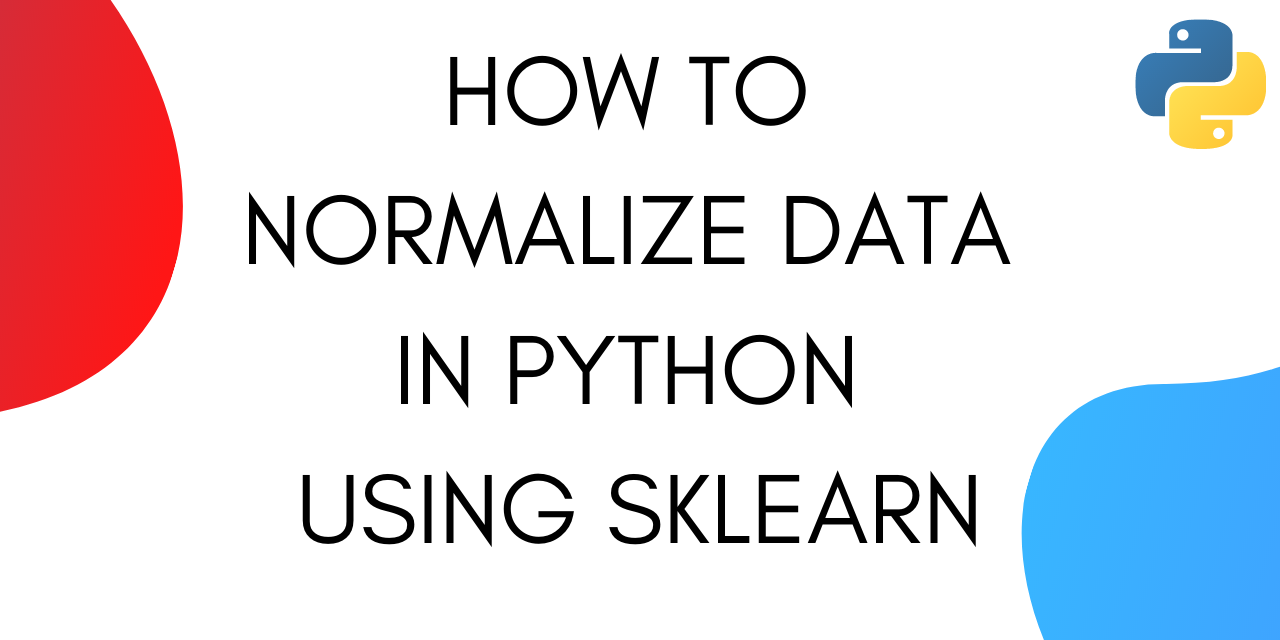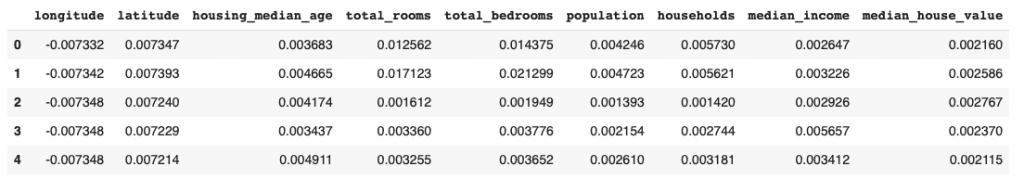# 2 Easy Ways to Normalize data in Python

Published on August 3, 2022By Jayant Verma
Developer and author at DigitalOcean.While we believe that this content benefits our community, we have not yet thoroughly reviewed it. If you have any suggestions for improvements, please let us know by clicking the “report an issue“ button at the bottom of the tutorial.

In this tutorial, we are going to learn about how to normalize data in Python. While normalizing we change the scale of the data. Data is most commonly rescaled to fall between 0-1.

## Why Do We Need To Normalize Data in Python?

Machine learning algorithms tend to perform better or converge faster when the different features (variables) are on a smaller scale. Therefore it is common practice to normalize the data before training machine learning models on it.

Normalization also makes the training process less sensitive to the scale of the features. This results in getting better coefficients after training.

This process of making features more suitable for training by rescaling is called feature scaling.

The formula for Normalization is given below :

We subtract the minimum value from each entry and then divide the result by the range. Where range is the difference between the maximum value and the minimum value.

## Steps to Normalize Data in Python

We are going to discuss two different ways to normalize data in python.

The first one is by using the method ‘normalize()’ under sklearn.

### Using normalize() from sklearn

Let’s start by importing processing from sklearn.

``````from sklearn import preprocessing
``````

Now, let’s create an array using Numpy.

``````import numpy as np
x_array = np.array([2,3,5,6,7,4,8,7,6])
``````

Now we can use the normalize() method on the array. This method normalizes data along a row. Let’s see the method in action.

``````normalized_arr = preprocessing.normalize([x_array])
print(normalized_arr)
``````

### Complete code

Here’s the complete code from this section :

``````from sklearn import preprocessing
import numpy as np
x_array = np.array([2,3,5,6,7,4,8,7,6])
normalized_arr = preprocessing.normalize([x_array])
print(normalized_arr)
``````

Output :

``````[0.11785113, 0.1767767 , 0.29462783, 0.35355339, 0.41247896,
0.23570226, 0.47140452, 0.41247896, 0.35355339]
``````

We can see that all the values are now between the range 0 to 1. This is how the normalize() method under sklearn works.

You can also normalize columns in a dataset using this method. Let’s see how to do that next.

### Normalize columns in a dataset using normalize()

Since normalize() only normalizes values along rows, we need to convert the column into an array before we apply the method.

To demonstrate we are going to use the California Housing dataset.

Let’s start by importing the dataset.

``````import pandas as pd
``````

Next, we need to pick a column and convert it into an array. We are going to use the 'total_bedrooms’ column.

``````from sklearn import preprocessing
x_array = np.array(housing['total_bedrooms'])
normalized_arr = preprocessing.normalize([x_array])
print(normalized_arr)
``````

Output :

``````[[0.01437454 0.02129852 0.00194947 ... 0.00594924 0.00618453 0.00336115]]
``````

### How to Normalize a Dataset Without Converting Columns to Array?

Let’s see what happens when we try to normalize a dataset without converting features into arrays for processing.

``````from sklearn import preprocessing
import pandas as pd
d = preprocessing.normalize(housing)
scaled_df = pd.DataFrame(d, columns=names)
``````

Output :

Here the values are normalized along the rows, which can be very unintuitive. Normalizing along rows means that each individual sample is normalized instead of the features.

However, you can specify the axis while calling the method to normalize along a feature (column).

The value of axis parameter is set to 1 by default. If we change the value to 0, the process of normalization happens along a column.

``````from sklearn import preprocessing
import pandas as pd
d = preprocessing.normalize(housing, axis=0)
scaled_df = pd.DataFrame(d, columns=names)
``````

Output :You can see that the column for total_bedrooms in the output matches the one we got above after converting it into an array and then normalizing.

### Using MinMaxScaler() to Normalize Data in Python

Sklearn provides another option when it comes to normalizing data: MinMaxScaler.

This is a more popular choice for normalizing datasets.

Here’s the code for normalizing the housing dataset using MinMaxScaler :

``````from sklearn import preprocessing
import pandas as pd
scaler = preprocessing.MinMaxScaler()
names = housing.columns
d = scaler.fit_transform(housing)
scaled_df = pd.DataFrame(d, columns=names)
``````

Output :

You can see that the values in the output are between (0 and 1).

MinMaxScaler also gives you the option to select feature range. By default, the range is set to (0,1). Let’s see how to change the range to (0,2).

``````from sklearn import preprocessing
import pandas as pd
scaler = preprocessing.MinMaxScaler(feature_range=(0, 2))
names = housing.columns
d = scaler.fit_transform(housing)
scaled_df = pd.DataFrame(d, columns=names)
``````

Output :

The values in the output are now between (0,2).

## Conclusion

These are two methods to normalize data in Python. We covered two methods of normalizing data under sklearn. Hope you had fun learning with us!Developer and author at DigitalOcean.

#### Still looking for an answer?

If we have a table xy and we have to “add a check if fields are normalized integral (|E|^2)=1…. “…What we have to do?

- Fani

In your example: [2,3,5,6,7,4,8,7,6] x_min = 2, x_max = 8, right? Then, according to your formula, the number 8 should turn into 1, and the number 2 into 0. But something is wrong) Here, normalization does not take place according to this formula, but simply each element is divided by the root of the sum of the squares of all elements.

- Alexander

Nice clear article - thanks for posting. The earlier code samples need the line: names = housing.columns

- Phil J